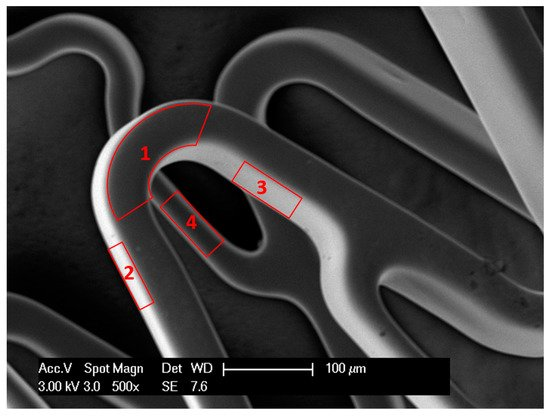Determination of the entire stent surface area by a new analytical method
Materials 13, 1 (2020).
M. Saqib, R. Bernhardt, M. Kästner, N. Beshchasna, G. Cuniberti, and J. Opitz.
Journal DOI: https://doi.org/10.3390/ma13245633

Stenting is a widely used treatment procedure for coronary artery disease around the world. Stents have a complex geometry, which makes the characterization of their corrosion difficult due to the absence of a mathematical model to calculate the entire stent surface area (ESSA). Therefore, corrosion experiments with stents are mostly based on qualitative analysis. Additionally, the quantitative analysis of corrosion is conducted with simpler samples made of stent material instead of stents, in most cases. At present, several methods are available to calculate the stent outer surface area (SOSA), whereas no model exists for the calculation of the ESSA. This paper presents a novel mathematical model for the calculation of the ESSA using the SOSA as one of the main parameters. The ESSA of seven magnesium alloy stents (MeKo Laser Material Processing GmbH, Sarstedt, Germany) were calculated using the developed model. The calculated SOSA and ESSA for all stents are 33.34%(±0.26%) and 111.86 mm (±0.85 mm), respectively. The model is validated by micro-computed tomography (micro-CT), with a difference of 12.34% (±0.46%). The value of corrosion rates calculated using the ESSA computed with the developed model will be 12.34% (±0.46%) less than that of using ESSA obtained by micro-CT.Share

Involved ScientistsDetermination of the entire stent surface area by a new analytical method
Materials 13, 1 (2020).
M. Saqib, R. Bernhardt, M. Kästner, N. Beshchasna, G. Cuniberti, and J. Opitz.
Journal DOI: https://doi.org/10.3390/ma13245633

Stenting is a widely used treatment procedure for coronary artery disease around the world. Stents have a complex geometry, which makes the characterization of their corrosion difficult due to the absence of a mathematical model to calculate the entire stent surface area (ESSA). Therefore, corrosion experiments with stents are mostly based on qualitative analysis. Additionally, the quantitative analysis of corrosion is conducted with simpler samples made of stent material instead of stents, in most cases. At present, several methods are available to calculate the stent outer surface area (SOSA), whereas no model exists for the calculation of the ESSA. This paper presents a novel mathematical model for the calculation of the ESSA using the SOSA as one of the main parameters. The ESSA of seven magnesium alloy stents (MeKo Laser Material Processing GmbH, Sarstedt, Germany) were calculated using the developed model. The calculated SOSA and ESSA for all stents are 33.34%(±0.26%) and 111.86 mm (±0.85 mm), respectively. The model is validated by micro-computed tomography (micro-CT), with a difference of 12.34% (±0.46%). The value of corrosion rates calculated using the ESSA computed with the developed model will be 12.34% (±0.46%) less than that of using ESSA obtained by micro-CT.